El enlace fue enviado a su email.

No se pudo enviar el enlace a su email. Por favor, verificar su correo electrónico.

Ayuda en línea

Tree
Settings
Producto:
Programa:
Idioma:

Shahunyants

The Shahunyants method is a general method of slices developed on the basis of limit equilibrium. It requires satisfying equilibrium of forces and moments acting on individual blocks. The blocks are created by dividing the soil above the slip surface by dividing planes. Forces acting on individual blocks are displayed in the following figure: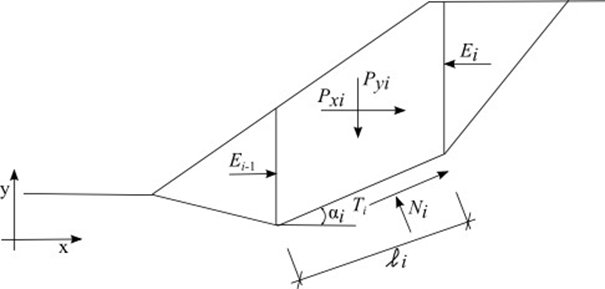Static scheme - Shahunyants method

Each block assumes action of the following forces:

 where: Pyi - resultant of vertical forces acting on a given block (block weight, block surcharge, earthquake, anchor forces, …) Pxi - resultant of horizontal forces on a given block (block surcharge, earthquake, anchor forces, geo-reinforcements, …) Ei+1, Ei - forces developed between blocks Ni - reaction below the block normal to the slip surface segment Ti - friction force on the slip surface segment αi - inclination of the slip surface segment li - length of the slip surface segment φi - angle of internal friction of soil on the slip surface segment ci - soil cohesion on the slip surface segment

The following assumptions are adopted in the Shahunyants method to calculate the limit state on a given block:

• dividing planes between blocks are always vertical
• slope of forces Ei acting between blocks is zero, forces act horizontally

Solution procedure:

Forces Pyi and Pxi are first transformed with the help of expressions (1) and (2) into directions of forces Ti and Ni. For a positive angle αi (the same way as in the schema) the force PNi acts in the direction opposite to Ni, the force PQi acts in the direction opposite to Ti.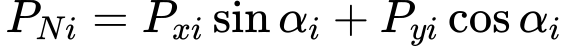(1)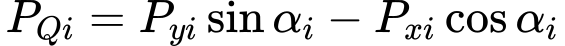(2)

The forces acting along the slip surface segment are related by: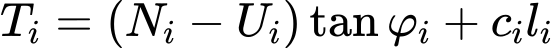(3)

 where: Ui - pore pressure on the slip surface segment

The force equations of equilibrium are fulfilled on the block:

The equilibrium condition in the direction normal to the slip surface segment: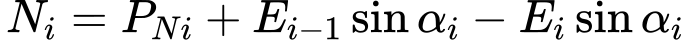(4)

The equilibrium condition in the direction parallel to the slip surface segment: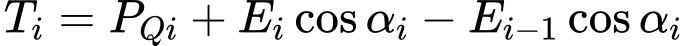(5)

Introducing Eq. (3) into Eq. (5) gives: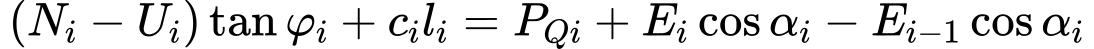(6)

Next, substituting Eq. (4) into Eq. (6) gives: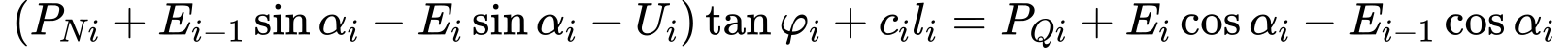(7)

After some algebra:(8)

Exploiting the following mathematical expression:(9)

yields Eq. (8) in the form:(10)

This can be modified as:(11)

to provide the recurrent expression for Ei forces acting between blocks as:(12)

At this stage the analysis enters the factor of safety Ku. The factor of safety is the value which bring the forces acting on individual blocks of soil into the state of limit states. This is achieved by multiplying active forces, i.e. forces contributing to sliding of the soil mass above the slip surface, by the factor of safety. Active forces are in Eq. (12) contained within the term PQi. This term contains on the one hand active forces that contribute to sliding and on the other hand forces that resist to sliding. The contributing forces will be denoted as PQi,sd whereas the resisting forces as PQi,ud. Eq. (12) then becomes:(13)

Providing the value of PQi is positive then it contributes to sliding and will be assumed as active force PQi,sd. Providing the value of PQi is negative then it resists to sliding and will be assumed as force PQi,ud. Therefore subtracting the value PQi,ud, which is negative, in Eq. (13) means essentially adding the positive value, so we can formally write:(14)

At the slip surface origin the value of E0 = 0. The value of E1 is then given by:(15)

The value of E2 is then given by:(16)

Similarly we may determine the values of all forces acting between blocks. It further holds at the end point of the slip surface we have En = 0. Exploiting the previous expressions this can be written as:(17)

This equation directly provides the factor of safety Ku in the form:(18)